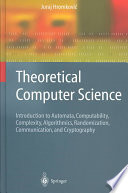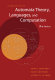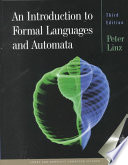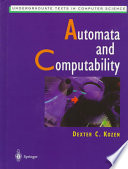### Introduction to Theoretical Computer Science

#### Course Description

The course introduces formal models of automata and grammars used for description, definition, software and hardware implementation, as well as verification of correctness of execution of computer and communication processes, protocols, and systems. Basic properties of computing processes and systems, such as determinism, decidability, computability, complexity, and tractability, are explained. Basics of automata theory, formal grammars, and languages are given. Chomsky hierarchy of languages is presented: regular languages, context-free languages, context-sensitive languages, recursive languages, and recursively-enumerable languages. Complexity classes and hierarchy of complexity classes are defined: complete and hard problems, polynomial classes P and NP, and reduction method.

#### Learning Outcomes

1. model the behavior of a computer system and communication protocol using formal language
2. model the behavior of a computer system, communication protocol or other kinds of technical systems using formal language
3. design a computing process using a formal model
4. apply formal models to verify the corectness of a computer system
5. categorize a problem with respect to Chomsky hierarchy of formal languages
6. select the optimal formal model for description, design, and implementation of a computer system
7. estimate time and space complexity of a computing process
8. evaluate the efficiency of a computer system and communication protocol
9. assess the problem complexity class

#### Forms of Teaching

Lectures

Lecturer-driven classroom presentations of theoretical concepts with examples and problem solving

Laboratory

Software implementation of finite automata, finite automata minimization algorithms, pushdow automata, and recursive descent parsing. Students individually implement given assignment in a recommended programming language or tool, and submit their solutions to automatic online evaluation.

Continuous Assessment Exam
Laboratory Exercises 50 % 30 % 50 % 0 %
Mid Term Exam: Written 50 % 35 % 0 %
Final Exam: Written 50 % 35 %
Exam: Written 50 % 100 %
Exam: Oral 100 %

#### Week by Week Schedule

1. String derivation and parsing, Deterministic finite automata (DFA)
2. Minimization of DFA, Nondeterministic finite automata (NFA and NFA with eps-transitions)
3. Equivalence of DFA, NFA, and NFA with eps-transitions
4. Regular languages, Regular expressions, Operations on automata, Properties of regular, context-free, recursive and recursively-enumerable languages, pumping lemma
5. Context-free languages, Properties of regular, context-free, recursive and recursively-enumerable languages, pumping lemma
6. Context-free grammar
7. Pushdown automata
8. Midterm exam
9. Recursively-enumerable languages, Turing machine, Unrestricted grammar, Properties of regular, context-free, recursive and recursively-enumerable languages, pumping lemma, Pumping lemma (proving languages non-regular and non-context-free)
10. Chomsky hierarchy of formal languages, grammars, and automata, Context-sensitive languages, context-sensitive grammar, and linear bounded automata
11. Definitions of space and time complexity, The halting problem, (Non)deterministic computation, Tractability, Computability with examples of uncomputable functions, Decidability with examples of undecidable functions
12. Introduction to P and NP classes, NP-complete and NP-hard problems, Introduction to the NP-complete class and exemplary NP-complete problems (e.g., SAT, Knapsack)
13. Review of the classes P and NP; Introduce P-space and EXP, Polynomial hierarchy, NP-completeness (Cook-Levin theorem), Classic NP-complete problems
14. Reduction Techniques, Complexity classes, Polynomial complexity classes (P and NP classes), Definition of complete and hard problems, P-complete (hard) and NP-complete (hard) problems
15. Final exam

#### Study Programmes

Computing (study)
(4. semester)

#### Literature

Siniša Srbljić (2010.), Uvod u teoriju računarstva, ElementJuraj Hromkovič (2003.), Theoretical Computer Science, Springer Science & Business MediaJohn E. Hopcroft, Rajeev Motwani, Jeffrey D. Ullman (2007.), Introduction to Automata Theory, Languages, and Computation, Addison-WesleyPeter Linz (2001.), An Introduction to Formal Languages and Automata, Jones & Bartlett LearningDexter C. Kozen (2007.), Automata and Computability, Springer Science & Business Media

#### General

ID 183412
Summer semester
5 ECTS
L1 English Level
L2 e-Learning
60 Lectures
10 Laboratory exercises